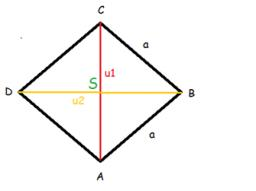# Circumference 4712

If you enlarge the side of the diamond by 4 cm, its circumference will be 20 cm. How long was the side of the original diamond?

a =  1

### Step-by-step explanation:

4·(a+4)=20

4a = 4

a = 4/4 = 1

a = 1

Our simple equation calculator calculates it.Did you find an error or inaccuracy? Feel free to write us. Thank you!

Tips for related online calculators
Do you have a linear equation or system of equations and looking for its solution? Or do you have a quadratic equation?

#### Grade of the word problem:

We encourage you to watch this tutorial video on this math problem: